Open in App
Not now

# Python | Pandas dataframe.rpow()

• Last Updated : 04 Jan, 2022

Python is a great language for doing data analysis, primarily because of the fantastic ecosystem of data-centric python packages. Pandas is one of those packages and makes importing and analyzing data much easier.
Pandas dataframe.rpow() function is used for finding the exponential power of dataframe and other, element-wise (binary operator rfloordiv). This function is essentially same as doing other ** dataframe but with a support to substitute for missing data in one of the inputs.

Syntax:DataFrame.rpow(other, axis=’columns’, level=None, fill_value=None)
Parameters :
other : Series, DataFrame, or constant
axis : For Series input, axis to match Series index on
level : Broadcast across a level, matching Index values on the passed MultiIndex level
fill_value : Fill existing missing (NaN) values, and any new element needed for successful DataFrame alignment, with this value before computation. If data in both corresponding DataFrame locations is missing the result will be missing.
Returns : result : DataFrame

Example #1: Use rpow() function to raise each element of a series to a corresponding value in a dataframe over the column axis.

## Python3

 `# importing pandas as pd` `import` `pandas as pd`   `# Creating the dataframe ` `df ``=` `pd.DataFrame({"A":[``1``, ``5``, ``3``, ``4``, ``2``],` `                   ``"B":[``3``, ``2``, ``4``, ``3``, ``4``], ` `                   ``"C":[``2``, ``2``, ``7``, ``3``, ``4``], ` `                   ``"D":[``4``, ``3``, ``6``, ``12``, ``7``]},` `                    ``index ``=``["A1", "A2", "A3", "A4", "A5"])`   `# Print the dataframe` `df`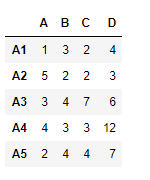Let’s create the series

## Python3

 `# importing pandas as pd` `import` `pandas as pd`   `# Create the series` `sr ``=` `pd.Series([``12``, ``25``, ``64``, ``18``], index ``=``["A", "B", "C", "D"])`   `# Print the series` `sr`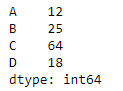Lets use the dataframe.rpow() function to raise each element in a series to the power of corresponding element in the dataframe.

## Python3

 `# equivalent to sr ** df` `df.rpow(sr, axis ``=` `1``)`

Output :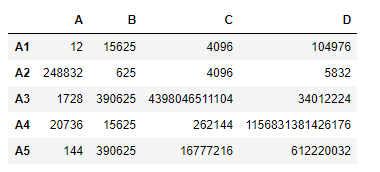Example #2: Use rpow() function to raise each element in a dataframe to the power of corresponding element in other dataframe

## Python3

 `# importing pandas as pd` `import` `pandas as pd`   `# Creating the first dataframe ` `df1 ``=` `pd.DataFrame({"A":[``1``, ``5``, ``3``, ``4``, ``2``],` `                    ``"B":[``3``, ``2``, ``4``, ``3``, ``4``],` `                    ``"C":[``2``, ``2``, ``7``, ``3``, ``4``],` `                    ``"D":[``4``, ``3``, ``6``, ``12``, ``7``]},` `                     ``index ``=``["A1", "A2", "A3", "A4", "A5"])`   `# Creating the second dataframe` `df2 ``=` `pd.DataFrame({"A":[``10``, ``11``, ``7``, ``8``, ``5``],` `                    ``"B":[``21``, ``5``, ``32``, ``4``, ``6``],` `                    ``"C":[``11``, ``21``, ``23``, ``7``, ``9``],` `                    ``"D":[``1``, ``5``, ``3``, ``8``, ``6``]}, ` `                     ``index ``=``["A1", "A2", "A3", "A4", "A5"])`   `# Print the first dataframe` `print``(df1)`   `# Print the second dataframe` `print``(df2)`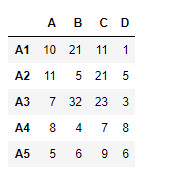Lets perform df2 ** df1

## Python3

 `# raise df2 to the power of df1` `df1.rpow(df2)`

Output :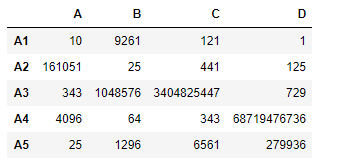My Personal Notes arrow_drop_up
Related Articles